##### The Japanese Maple specialist
Direct order Contact Help / Services Newsletter# Shelf to displaySearch

Japanese MaplesYoung maples and bonsaiAcer seeds and more Bonsai soil and fertiliserVarieties introduced into EuropeHostasWind bells and chimesSaxifragesSpecial offer

Packing and shipping charges

# Fujinami nishiki

Yama momiji fujinami nishiki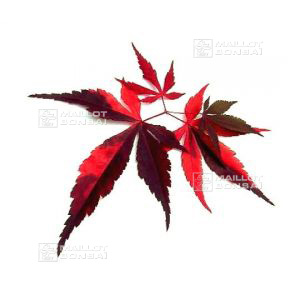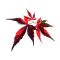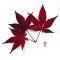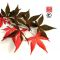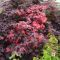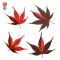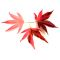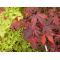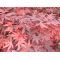ref. : 349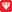Choose the right size
Pot size

This item is temporarily unavailable

###### Description

Leaves: crimson in spring, becoming variegated with shiny red. The colour gets bolder in May, June and July. The autumn colour is purplish red. A unique, very impressive colour. Variety as yet only very rarely grown. First introduced in Europe by Guy Maillot.

Small size delivered trees about 20/25 cm height.

Be careful trees potted in spring 2019 don't touch the root ball before spring 2020.

#colour 3.6 #spring 3.6 #fujinami 2.8 #amoenum 2.7 #nishiki 2.7 #trees 2.5 #acer 2.4 #very 2.4 #variegated 2 #impressive 2

###### Technical description
 Species Acer amoenum same species items Variety Yama momiji fujinami nishiki Leaf colour Variegated leaves same colour items Leaf shape Palmated same shaped items Adult height Between 2 and 4 meters same height items Port Natural same port items Expositions Sun same exposition items

Formule
(( ROUND((CHAR_LENGTH(b.article_nom)-CHAR_LENGTH(REPLACE(b.article_nom, 'spring', '')))/LENGTH('spring')) + ROUND((CHAR_LENGTH(b.article_description)-CHAR_LENGTH(REPLACE(b.article_description, 'spring', '')))/LENGTH('spring')) ) * 3.6) + (( ROUND((CHAR_LENGTH(b.article_nom)-CHAR_LENGTH(REPLACE(b.article_nom, 'colour', '')))/LENGTH('colour')) + ROUND((CHAR_LENGTH(b.article_description)-CHAR_LENGTH(REPLACE(b.article_description, 'colour', '')))/LENGTH('colour')) ) * 3.6) + (( ROUND((CHAR_LENGTH(b.article_nom)-CHAR_LENGTH(REPLACE(b.article_nom, 'fujinami', '')))/LENGTH('fujinami')) + ROUND((CHAR_LENGTH(b.article_description)-CHAR_LENGTH(REPLACE(b.article_description, 'fujinami', '')))/LENGTH('fujinami')) ) * 2.8) + (( ROUND((CHAR_LENGTH(b.article_nom)-CHAR_LENGTH(REPLACE(b.article_nom, 'nishiki', '')))/LENGTH('nishiki')) + ROUND((CHAR_LENGTH(b.article_description)-CHAR_LENGTH(REPLACE(b.article_description, 'nishiki', '')))/LENGTH('nishiki')) ) * 2.7) + (( ROUND((CHAR_LENGTH(b.article_nom)-CHAR_LENGTH(REPLACE(b.article_nom, 'trees', '')))/LENGTH('trees')) + ROUND((CHAR_LENGTH(b.article_description)-CHAR_LENGTH(REPLACE(b.article_description, 'trees', '')))/LENGTH('trees')) ) * 2.5) + (( ROUND((CHAR_LENGTH(b.article_nom)-CHAR_LENGTH(REPLACE(b.article_nom, 'very', '')))/LENGTH('very')) + ROUND((CHAR_LENGTH(b.article_description)-CHAR_LENGTH(REPLACE(b.article_description, 'very', '')))/LENGTH('very')) ) * 2.4) + (( ROUND((CHAR_LENGTH(b.article_nom)-CHAR_LENGTH(REPLACE(b.article_nom, 'variegated', '')))/LENGTH('variegated')) + ROUND((CHAR_LENGTH(b.article_description)-CHAR_LENGTH(REPLACE(b.article_description, 'variegated', '')))/LENGTH('variegated')) ) * 2) + (( ROUND((CHAR_LENGTH(b.article_nom)-CHAR_LENGTH(REPLACE(b.article_nom, 'introduced', '')))/LENGTH('introduced')) + ROUND((CHAR_LENGTH(b.article_description)-CHAR_LENGTH(REPLACE(b.article_description, 'introduced', '')))/LENGTH('introduced')) ) * 2) + (( ROUND((CHAR_LENGTH(b.article_nom)-CHAR_LENGTH(REPLACE(b.article_nom, 'impressive', '')))/LENGTH('impressive')) + ROUND((CHAR_LENGTH(b.article_description)-CHAR_LENGTH(REPLACE(b.article_description, 'impressive', '')))/LENGTH('impressive')) ) * 2) + (( ROUND((CHAR_LENGTH(b.article_nom)-CHAR_LENGTH(REPLACE(b.article_nom, 'delivered', '')))/LENGTH('delivered')) + ROUND((CHAR_LENGTH(b.article_description)-CHAR_LENGTH(REPLACE(b.article_description, 'delivered', '')))/LENGTH('delivered')) ) * 1.9)

## Secure payment## Delivery

Our logistic partners :04 74 55 23 48
Pépinière MAILLOT-BONSAÏ
Le Bois Frazy
01990 RELEVANT - FRANCE
on appointment